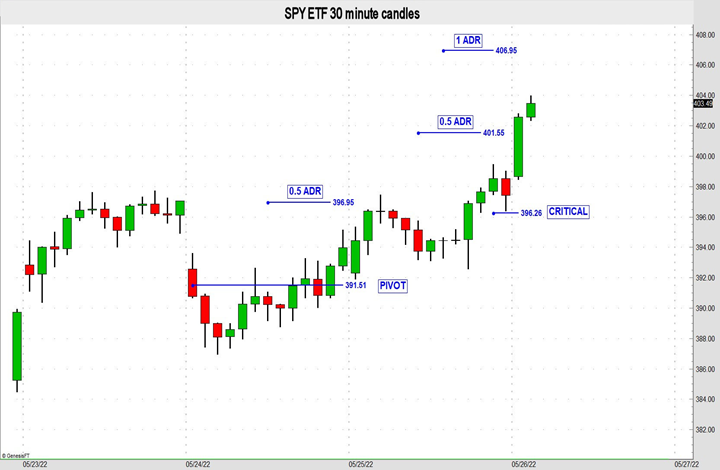# How to Use Critical or Pivotal Prices

Critical or pivotal prices are used in various ways. Some traders use them to identify support and resistance areas, entry and exit zones. They may also be used to define momentum. There are more than a few ways to determine daily pivot points. The most popular requires some basic math using the high, low and close; sometimes the open price is included in the equation.

###### Pivot Calculation With Support/Resistance

The most common way to calculate a daily pivot is to find the sum of the high, low and close of the day, and then divide by 3. From that point we can calculate a first support and first resistance level. To find first support multiply the pivot point (PP) by 2, and then subtract the high price. To find first resistance double the pivot minus the low price. For example, SPY high=398, low=392, close=394, and then pivot = 394.7. First resistance would then be (394.7 x 2) – 392 (low) = 395.4. First support would be 391.4, which is 2 x PP minus high.

###### Pivot Points Combined With ATR

One of my favorite ways to define support/resistance levels is to first calculate a daily pivot, and then calculate an average day range (ADR) using the previous 14 days. Most charting packages have an indicator called average true range (ATR); use this to measure average ranges for any time frame. To plot resistance 1 (R1) start from the pivot and add 50% of an ADR. To get second resistance (R2) add 100% of an ADR to the pivot price. Conversely, to identify support (S1 & S2) levels subtract 0.5 ADR from the pivot to get S1 and 1 ADR to mark S2.

Of course, this technique can be used to fit your preferred trading style. For longer-term trades I use the weekly high, low and close to find the pivot. Use a 9-week average true range (AWR) to determine S1 and S2, and then subtract 0.5 AWR and 1 AWR from the pivot, respectively. Or add 0.5 and 1 AWR to the pivot to plot R1 and R2. The longer-term support/resistance levels are useful for defining price targets or strikes as well.

###### Critical Prices vs. Pivots

Pivots are usually measured while critical prices are observed. Sometimes the two are similar. I prefer to use intraday charts to identify critical areas that I believe are more reliable for defining support/resistance. One type of critical price is the low dip following a breakout higher through a trendline or channel or the high bounce following a breakout lower.

The graph below illustrates an example of both pivotal and critical levels from which resistance levels were calculated. The pivot on 5/24 was 391.51. Measuring 0.5 ADR higher from that point is R1 and that level did provide resistance when tested the next session. The critical point or low dip late in the day (5/25) was 396.26. The upside objectives are shown as 0.5 ADR and 1 ADR.Set Goals With Pivots

After a violation of a flag or pennant formation is realized, you should start measuring using ATRs to set objectives. Projecting targets from a pivot or critical price is a great way to take incremental profits and/or trail stops to lock in potentially more profit.

John Seguin
Senior Technical Analyst
Market Taker Mentoring, Inc.

## 1 reply on “How to Use Critical or Pivotal Prices”harold wargosays:

I love following MTM and Dan P. Im still wainting for the books I ordered 2/22/22. If I don’t get the 3 books, not a big deal.